Excel Multiplication Table Practice Workbook

Written by Debra Dalgleish from Contextures Blog

Do you remember the number grids from your schools days, where you filled in the multiplication answers? What’s 4 times 8? Or 9 times 7?  If you need a refresher, or you’re helping someone learn them, I’ve got an Excel multiplication table practice workbook for you.

Studying at Home

Those times tables came back to haunt me this year, when the schools closed because of the pandemic.

Students were working from home, so I had Zoom meetings with my grandson, and helped  with his math homework. We did lots of work on those times tables!

Excel Multiplication Workbook

The school year has ended now, but maybe my grandson wants to practise his times tables all summer! (or not)

Just in case, I’ve made an Excel multiplication table practice workbook, and you can download a copy too.

There’s a link near the end of this blog post, and there are a couple of videos too, with times table memorization tips.

Times Table Practice – One Row

There are 2 practice sheets in the Excel multiplication workbook.

On the OneRow practice sheet, pick a number, from 1 to 12, from a drop down list.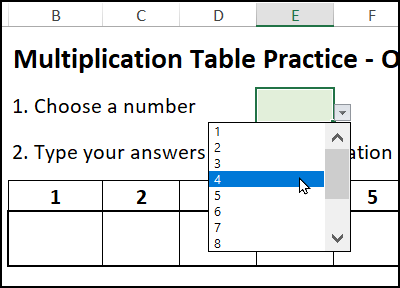Then, fill in the grid with the multiplication answers – or your best guess!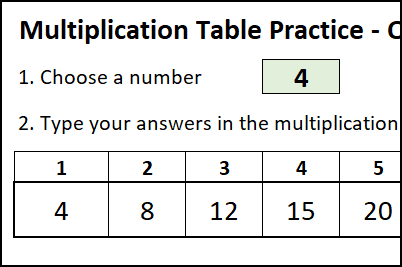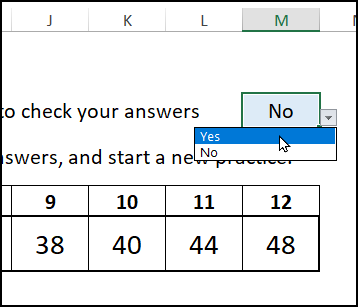Wrong answers are highlighted with orange fill colour. Uh oh, time to do another practice!Times Table Grid

On the other practice sheet, named Table, you’ll choose a range of numbers that you want to practise.

Rows are created for that range of numbers, where you can fill in the multiplication answers.Then, select “Yes” from the Check drop down, to highlight any errors.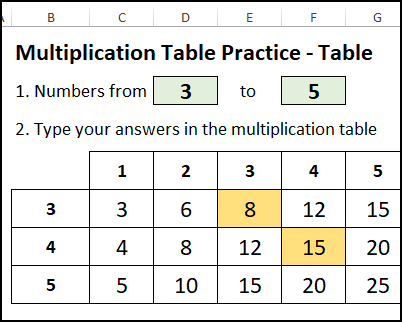Here’s the formula for the Conditional Formatting rule, to highlight wrong answers on the Table sheet.

=AND(C9<>””, \$M\$4=”Yes”, C9<>\$B9*C\$8)

Cell E9 has orange fill because 3×3=9, and the answer was typed as 8.

NOTE: Cell M4 has the drop down list, where you choose “Yes” to check your answers.To keep the Table sheet from looking cluttered, cell borders are only shown for the selected range of numbers.

That’s done with conditional formatting, using this formula:

=\$B9<>””

There are no grid borders in row 12, because cell B12 is empty.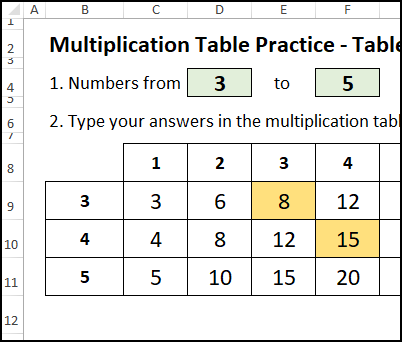Number Sequence

In column B, the multiplier numbers are shown, based on the range that you selected.

In cell B9, a formula links to cell D4, where you enter the first number in the range

=D4

To create the sequence, this formula is entered in B10, and copied down to B20:

=IF(B9=””,””, IF(B9=\$F\$4,””, SUM(B9,1)))

The result is an empty string (“”) if:

• the cell above has an empty string
• the cell above has a number equal to the end number in cell F4

Otherwise, the result is the number above, plus 1

In this example, the range is 2 to 6.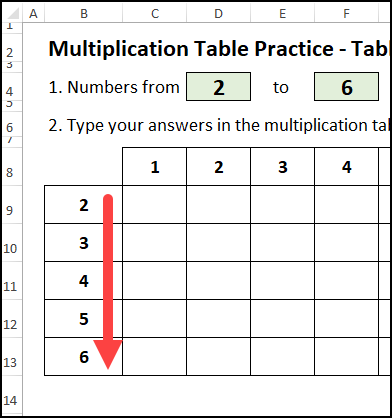Sheet Protection

In the sample file, the OneRow and Table sheets are protected, without a password

On those sheets,

• data entry cells are unlocked
• all the other cells are locked
• you can select the unlocked cells only

That helps protect the worksheet formulas, so they aren’t accidentally deleted.

To unprotect a sheet:

• On the Excel Ribbon, click the Review tab
• In the Protect group, click Unprotect Sheet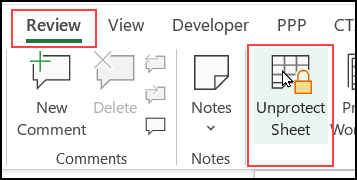Get the Sample File

To get the Excel Multiplication Table Practice workbook, go to the sample files page on my Contextures site.

In the Functions section, look for FN0065 – Excel Multiplication Tables.

The zipped Excel file is in xlsx format, and does not contain any macros.

If you’re helping someone learn the times tables too, the tips and tricks in these two videos might help.

I was glad to see that they’re still teaching the “Magic of 9s”, which we learned long ago.  It’s mentioned in both videos below.

How to Easily Memorize the Multiplication Table

This video  is aimed at parents and teachers, and covers the basics for teaching the times tables.

Fastest Way to Learn Multiplication Facts

This video is is geared for the students, and shows how some of the times tables tricks work.

___________________

Excel Multiplication Table Practice Workbook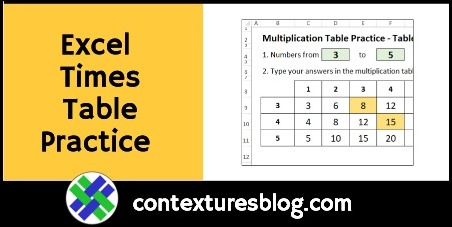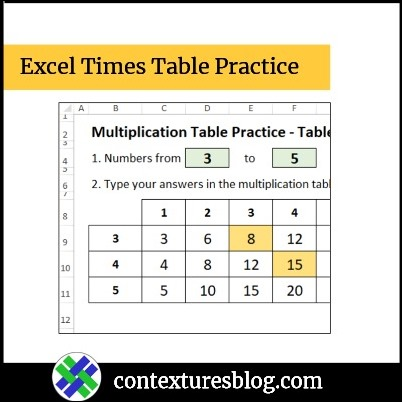___________________

Excel Multiplication Table Practice Workbook is a post from Contextures Blog and is not allowed to be copied to other sites